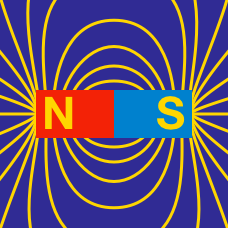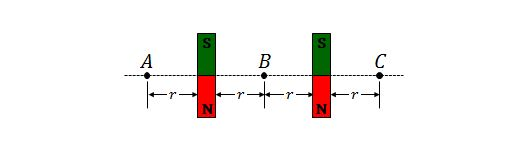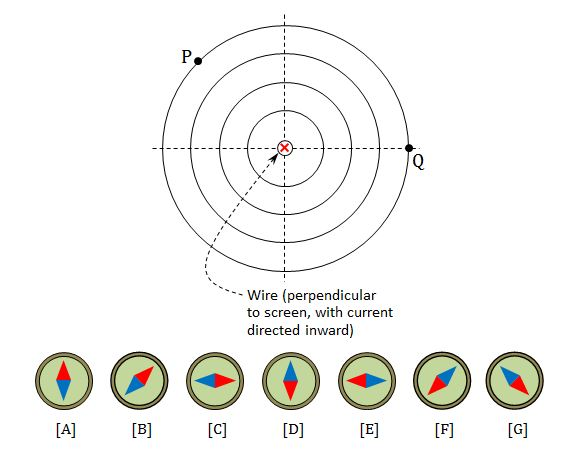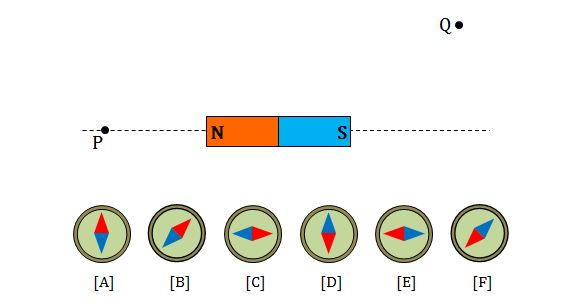Electricity and Magnetism

# Magnetic field linesTwo identical magnetic dipoles are both pointing straight up and down, and are separated by some distance, as shown above. Which of the following is the appropriate comparison of the magnitude of the magnetic field strength?The above diagram depicts a wire which is perpendicular to the screen, with current directed inward. If we put a magnetic portable compass at each point P and Q, which of the following are the appropriate directions of the compasses at points P and Q, respectively?

Neglect the Earth's magnetic field.

Assumptions and Details

• Red represents the north pole, blue the south.

Two current-carrying-wires are both pointing straight up and down, and are separated by some distance. If the directions of the two currents are parallel, what would the resulting magnetic field look like?

The symbol $\odot$ means the direction of the current is pointed out of the plane in the figure.If we put a magnetic portable compass at each point P and Q in the above diagram, which of the following are the appropriate respective directions of the two compasses?

Neglect the Earth's magnetic field.

Two magnetic dipoles are both pointing straight up and down, and are separated by some distance. The magnetic north pole of one magnetic dipole is at the top and that of the other is at the bottom. What would the resulting magnetic field look like?×